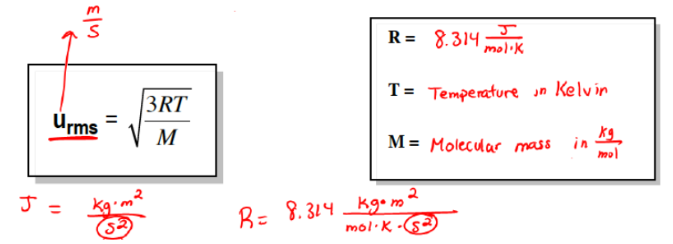# Problem: Calculate the root mean square velocity of F2,Cl2, and Br2 at 302 K .

###### FREE Expert Solution
• Root mean squared or RMS can be calculated using the provided temperature and the molar mass of the gas where:• We need the molar mass in kg/mol instead of g/mol because we have a unit J which can be simplified as kg2•m2•s-2
• Molar mass of F2, Cl2 and Brin kg/mol will appear as:

1 g = 1x10-3 kg/mol

MM of F2 : 19.0 (2) = 38 g/mol or 38x10-3 kg/mol

MM of Cl2 : 35.45 (2) = 70.90 g/mol or 70.90x10-3 kg/mol

MM of Br2 : 79.90 (2) = 159.8 g/mol or 159.8x10-3 kg/mol

85% (366 ratings)###### Problem Details

Calculate the root mean square velocity of F2,Cl2, and Br2 at 302 K .